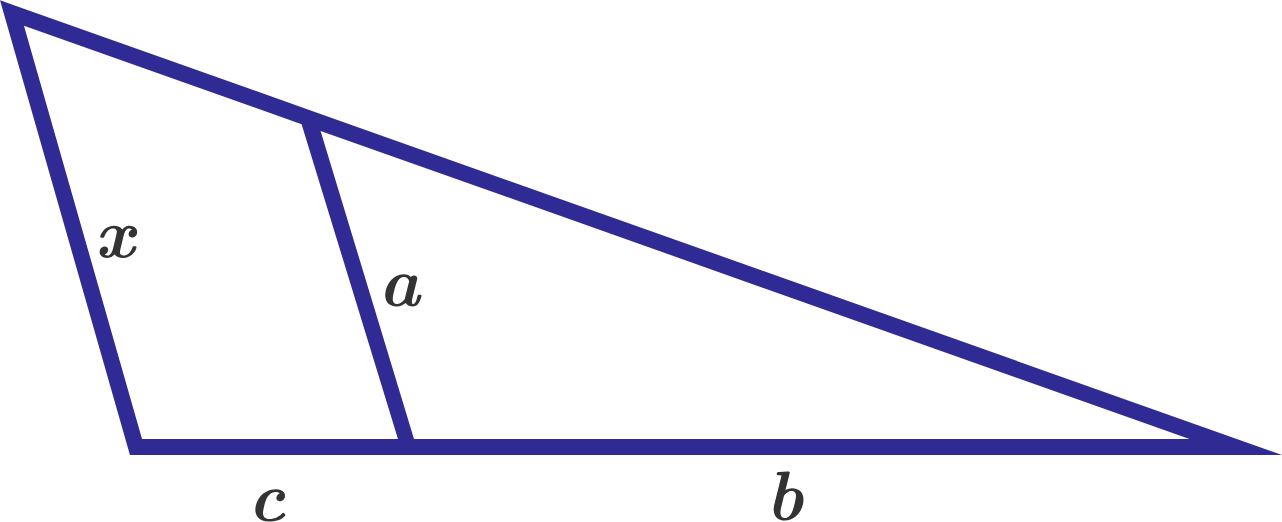# Familiar with similar?If the line segment with length $a$ is parallel to the line segment with length $x$ In the diagram above, then what is the value of $x?$

×